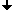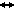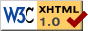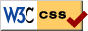Nonlinear Dynamics at the Free University Berlin

Winter 2009/2010

Forschungsseminar Dynamische Systeme

(Seminar für Diplomanden und Doktoranden)

(Internes Seminar der Arbeitsgruppe Nichtlineare Dynamik)

Prof. Dr. Bernold Fiedler

Juliette Hell, Dr. Stefan Liebscher

Program

 Oct 22, 2009 Orkan Konc The Pascal Triangle as a Dynamical System Nov 5, 2009 Alexander Slepukhin(St.Petersburg State University) Non-autonomous Global Attractors Nov 12, 2009 Prof. Dr. Sergey Yu. Pilyugin(St.Petersburg State University) Orbital shadowing property for diffeomorphisms We show that the C1-interior of the set of diffeomorphisms having the orbital shadowing property coincides with the set of structurally stable diffeomorphisms. Nov 19, 2009 Alexander Slepukhin(St.Petersburg State University) Introduction to the nonintegral dimension theory For some sets which have complex structure (like fractals), the usual concept of dimension may be not sufficient. We will introduce concepts of noninteger dimensions such as fractal and Hausdorff dimensions and consider their properties, relations between them, and methods to calculate them. Then we will study the behaviour of the fractal and Hausdorff dimensions on spaces which have Cartesian product structure. It is useful because the phase space of extended dynamical systems corresponding to non-autonomous differential equations has the Cartesian product structure. At the end, we will apply this theory for estimating the dimension of non-autonomous global attractors arising in the problem under consideration. Nov 26, 2009 Prof. Dr. Xiaojing Yang(Tsinghua University, Beijing) Boundedness and unboundedness of solutions of second order differential equations In this talk, I shall first give a general introduction to the boundedness and unboundedness problem of solutions of second order nonlinear ordinary differential equation. Then I give a simple description of the ideas of proving the boundedness and unboundedness problems of solutions. Last, I formulate some open questions and future research problems. Dec 10, 2009 Saul Ares(MPI Physics of Complex Systems, Dresden), Luis Morelli(University of Buenos Aires, Argentina) Discrete and continuum description of non-identical coupled phase oscillators with delayed coupling Spatiotemporal patterns can arise in arrays of interacting oscillators. Due to intrinsic lags in signal production, propagation, and processing, the interaction between oscillators is not instantaneous. In cellular systems, for example, slow complex kinetics introduce a time delay in intercellular coupling. Using a generic description based on coupled phased oscillators, we study the effects of delayed coupling on patterning and timing. We derive a continuum description for a system of coupled phase oscillators in which the coupling between neighboring cells is arbitrarily delayed. We show that delays introduce a renormalization of the coupling strength and autonomous frequencies of individual oscillators. We find analytical solutions for the phase profile and collective frequency in both discrete and continuum descriptions, and derive the stability threshold for discrete solutions. Motivated by a biological system, we discuss the open challenges of introducing a spatial drift in the problem: together with the delay, this introduces effective non-local coupling and exotic boundary conditions. Dec 17, 2009 Johannes Buchner Inhomogeneous cosmologies and the BKL-conjecture n mathematical cosmology, one of the big open questions is the structure of the inital singularity (i.e. the big bang). According to a conjecture of Belinskii, Khalatnikov and Lifshitz (BKL) from the 1970s, the approach is vacuum dominated, local and oscillatory (labeled "Mixmaster"). This "BKL-picture" is supported by many heuristics and numerical calculations, but mathematical proofs exist only in very special cases, like in spatial homogeneous spacetimes (where the Einstein Field Equations reduce to ODEs) and even here the picture is not complete. I will present some ideas how to tackle the problem in the spatially inhomogeneous case with less but still some symmetry - e.g. Gowdy and G2-models that lead to 1-dimensional PDEs. Furthermore, I will discuss the question if the formation of spikes (i.e. unbounded growth of spatial derivatives in the approach to the initial singularity) is consistent with the BKL-picture or not. Dec 18, 2009 10-12 a.m. Prof. Dr. Alan Rendall(MPI Gravitationsphysik, Potsdam) Introduction to Black Holes This talk is an elementary introduction to black holes. First I review some background from Newtonian physics. Then I discuss the Schwarzschild solution of the vacuum Einstein equations which represents a non-rotating, uncharged black hole. Then I briefly describe the Kerr and Reissner-Nordström solutions which represent rotating and charged black holes, respectively. I explain some conceptual and technical difficulties involved in the question of the dynamical stability of these solutions. Finally, I say something about the formation of black holes from regular initial data. Jan 7, 2010 Christian Kuehn(Cornell University) Multiple time-scale dynamics: bifurcations and mixed-mode oscillations First, we will give an introduction to fast-slow systems. The geometric viewpoint of the theory will be emphasized. Then we discuss the three-dimensional FitzHugh-Nagumo (FHN) equation and its bifurcations. The singular limit bifurcation diagram of the FHN equation will be derived. We shall also look at mixed-mode oscillations (MMOs) in the FHN equation and outline the role of MMOs in chemistry, neuroscience and physics. Jan 14, 2010 Prof. Dr. Arnd Scheel(University of Minnesota) Coherent structures and Liesegang pattern formation Liesegang rings form in strikingly simple experimental setups. The resulting patterns, sometimes regular, sometimes irregular, are ubiquitous in nature - yet mathematically poorly understood. We present models and results that lead to a conceptual understanding of existence and creation of such patterns, based on existence, stability, and bifurcation results for coherent structures in our models. A crucial role is played by pushed and pulled fronts in spinodal decomposition scenarios. Jan 21, 2010 Eyal Ron Traveling 2D waves in the Ares-Morelli Model The Ares Morelli model is a mathematical Kuramoto based model that describes development of vertebrates. It was presented to us in a seminar talk in December 2009. After that talk, Prof. Fiedler proved existence of traveling wave solutions to a one-dimention Ares-Morelli model. Based on Prof. Fiedler's proof, I will present traveling wave solutions to the Ares Morelli model on a 2D square lattice that supports a plane frequency profile. In addition, a 2D hexagonal lattice with radial frequency profile will be discussed, as it is a better approximation to reality.

Time and Place

Talks usually take place on Thursday at 2:15 p.m.
at the Free University Berlin
Room 140, Arnimallee 7, 14195 Berlin.

Guests are always welcome !

ArchiveLast change: Jan. 18, 2010
 This page strictly conforms to the XHTML1.0 standard and uses style sheets.